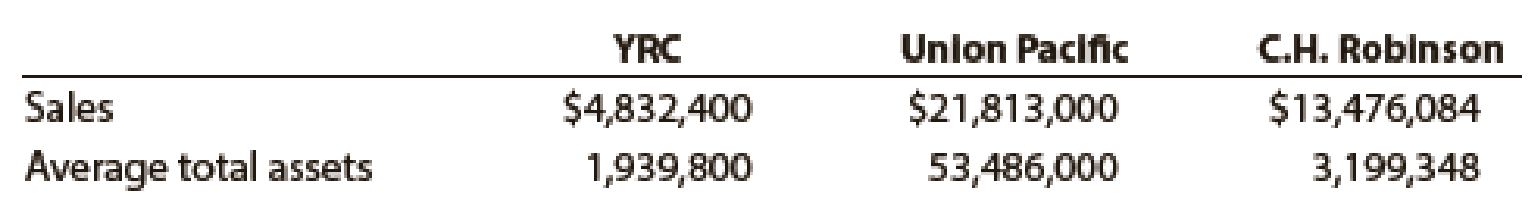Chapter 17, Problem 16E

Chapter
Section
Textbook Problem
1 views

# Asset turnoverThroe major segments of the transportation industry arc motor carriers such as YRC Worldwide, railroads such an Union Pacific, and transportation logistics service such as C.H. Robinson Worldwide, Inc. Recent financial statement information for these three companies follows (in thousands):a. Determine the asset turnover for all three companies. Round to one decimal place. b. Assume that the asset turnover for each company represents its respective industry segment. Interpret the differences in the asset turnover in terms of the operating characteristics of each of the respective segments.

(a)

To determine

Compute asset turnover ratio for all three companies.

Explanation

Financial Ratios: Financial ratios are the metrics used to evaluate the liquidity, capabilities, profitability, and overall performance of a company.

Asset turnover ratio is used to determine the asset’s efficiency towards sales. Asset turnover ratio is determined by dividing sales and average total assets.

Formula:

Asset turnover ratio = SalesAverage total assets

Compute asset turnover ratio for Y.

Asset turnover ratio = SalesAverage total assets=$4,832,400$1,939,800=2.5

Compute asset turnover ratio for U

(b)

To determine

Interpret the differences in asset turnover.

### Still sussing out bartleby?

Check out a sample textbook solution.

See a sample solution

#### The Solution to Your Study Problems

Bartleby provides explanations to thousands of textbook problems written by our experts, many with advanced degrees!

Get Started